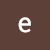# Program to add two binary numbers using bin function

Program to add two binary numbers using bin function.

A program that combines two binary numbers

In the following program, we use two built-in functions int () and bin ().

The int () function takes into account the source value provided by the given string‌, in the following example we convert the string (which is a binary value) to an integer, so we set the source value to 2 (binary numbers have base 2, decimals have a base value of 10).

After converting strings to integer values. we add them and the result is converted to binary number using bin () function.

Originally published at https://www.epythonguru.com.

--

--

# Find the Sum of the series: 1+1/2+1/3..+ 1/N in Python

In this, you will learn how to write a Python program to find the Sum of the series: 1 + 1/2 + 1/3 +… .. + 1 / N.

1. Take the number of terms to find the sum of the series.

2. Sum is initialized to zero.

3. Use a for loop to find the sum of the series and increment number by one at every iteration.

4. Print the whole series after rounding it to three decimal places.

# Let’s take a look at the code how to find the sum of series in Python Programming.

`enter number of terms:7 ['1/1', '1/2', '1/3', '1/4', '1/5', '1/6', '1/7'] sum of series is: 2.593`

Originally published at https://www.epythonguru.com.

--

--

# Python Program to Solve Quadratic equation

In this program, you will learn how to calculate the roots of a square equation when the coefficients a, b and c are known.

The quadratic formula uses “a”, “b” and “c” from

where “a”, “b” and “c” are just numbers; They are the “numerical modules” of the square equation they have given you to solve.

To understand the following program, you must have the knowledge to follow Python concepts:

Introduction to Python